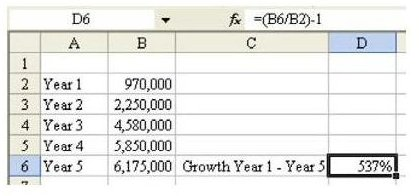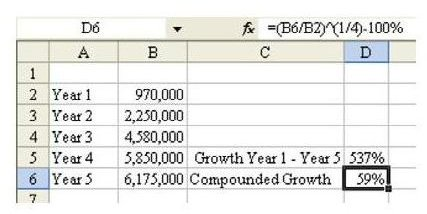# How to Calculate Growth Rate in Excel – Tips and Help from Mr. Excel

## Annual Growth Rate

The object of this Excel lesson is to explain how to use the spreadsheet application to find a compounded growth rate. One of the easiest ways to do this is use a working example, so we'll start out with the following scenario.

Problem: You work for a fast growing company. In the first year, they had \$970,000.00 in sales. In the fifth year, they had \$6,175,000.00 in sales. What was the compounded annual growth rate?

Now, we'll move on to showing how to solve this problem in Excel.

## Using Excel to Determine Compounded Growth Rate

Strategy: Sales in the fifth year are 6175/970 higher than the first year. The formula for growth is (year 5/year 1) – 100 percent. So, as shown in Fig. 443, the growth rate is 537 percent. (Click any of the images below for a larger view.)However, a compounded growth rate is a number, x, that will calculate out like this:

Year 1 * (100% + x) *(100% + x) *(100% + x) * (100% + x)= Year 5

This is the same as: Year 1 * (100% + x)^4 = Year 5

So, in order to calculate x, you have to be able to find the fourth root of (Year5/Year1). The formula to find the fourth root is to raise the number to the (1/4) power. Thus, as shown in Fig. 444, the formula to calculate the compounded growth rate is:

(Year 5/Year 1)^(1/4)–100% = xThe compounded growth rate is 59 percent.

Summary: Compounded growth rates are common calculations that require you to raise a number to a fractional power.

For similar tips, see 91 Tips for Calculating with Microsoft Excel. Written by Bill Jelen (also known as Mr. Excel), this collections of short, easy tutorials shows how to solve a variety of different problems in Excel – ranging from common calculations to more complex scenarios.